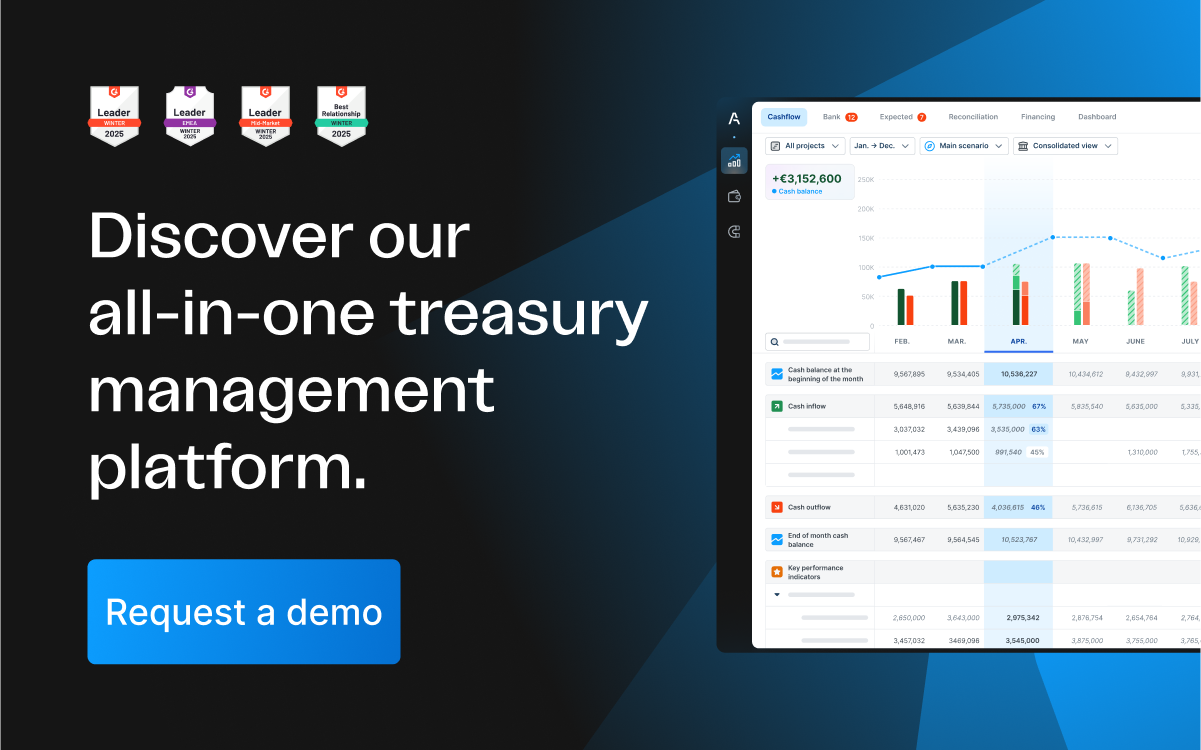# How leverage is used to improve a company's cash flowThe leverage effect is exploited by companies or investors to make promising investments with the help of debt capital, from which they expect higher revenues or returns. Here we show you exactly how it works, what the risks are and how to calculate it.

## Leverage: Definition

Leverage is generally understood to be the effect of using debt to make an investment, which in turn is intended to increase income or return.

The leverage effect can work in both directions: upwards or downwards. If the investment generates income or a positive return, this is multiplied by the leverage effect, so that more funds are available to repay the borrowed capital and at the same time surpluses are generated that can be invested further.If, on the other hand, the investment does not generate any income or even incurs costs, or if a negative return is generated, these are also amplified by the leverage effect: since the borrowed capital must be repaid, money must be made available from another source in order to repay the debt.

Companies use the leverage effect to finance their assets. In doing so, they raise debt capital with which they make investments (e.g. modernise their production facilities or expand). If the investments contribute to an increase in turnover, the company generates a higher profit without having used its equity capital.This enables the company to repay the loan and build up further reserves, thereby increasing its equity capital.

### Leverage in finance

Investors exploit the leverage effect in a similar way to companies. They borrow money and invest it on the capital market or in other assets (e.g. real estate). Provided that the assets develop positively in their value, investors can generate high returns in this way, even if they do not have enough equity to finance themselves.

## Leverage formula

There are several formulas for calculating leverage, which take different aspects into account. The ratio between two variables is always taken into account and is then referred to as the leverage ratio.

### Leverage ratio

#### Debt-to-assets ratio

If you compare the total debt with the total assets of a company, you get the debt-to- assets ratio:

Debt-to-assets ratio = Total debt / total assets x 100

Multiplying by 100 gives the ratio in percent, which indicates what percentage of the assets were financed with debt.

#### Debt-to-equity ratio

If you compare total debt with total equity, you get the debt-to-equity ratio:

Debt-to-equity ratio = Total debt / total equity x 100

This value indicates how high the share of debt capital is compared to equity capital. The higher this value, the more a company is financed by debt capital.

#### Debt-to-EBITDA ratio

The debt-to-EBITDA ratio indicates how high the revenue is in relation to the debt:

Debt-to-EBITDA ratio = Total debt / EBITDA x 100

The higher this value, the more leverage a company has, as its debts are very high in relation to its revenues.

## Leverage: Example

An IT company wants to expand its business model and offer server hosting. To do this, it needs its own data centre, server hardware and more staff to take care of maintenance and support. For the investment £500,000 is necessary. However, as the company does not have enough equity, it takes out a loan.

This loan represents financial leverage that enables the company to expand and thus opens up the opportunity to increase its revenues in the long term. On the other hand, it also creates a risk for the company, because if the investment does not generate the planned additional income, it will have difficulties repaying the loan.

At the end of the business year, it shows the following values in its balance sheet:

• Total debt: £500,000
• Total assets: £900,000
• Total equity: £100,000
• EBITDA: £200,000

We now calculate the different leverage ratios: Debt-to-assets ratio = £500,000 / £900,000 x 100 = 55.56% Debt-to-equity ratio = £500,000/ £100,000 x 100 = 500%. Debt-to-EBITDA ratio = £500,000 / £200,000 x 100 = 250%.

We can see here that the company has financed 55.56% of its assets with debt. Moreover, the debt is 5 times higher than the equity (500%), and the debt is 2.5 times higher (250%) than the income. The company's leverage is therefore very high.

If the investment proves to be lucrative, the leverage effect over time leads to an increase in turnover, which would not have been possible without borrowing. However, if revenues remain low and below expectations, the company will have problems repaying the loan.

## Leverage & its meaning for businesses

As we have seen from the different leverage ratios and the example above, the leverage effect can be positive or negative for a company. It all depends on whether the investment will perform as expected and generate a positive return.

Thus, leverage can boost a company because it can make investments for which it does not have enough equity, but it can also bankrupt it. Before borrowing, therefore, a thorough analysis must be made of whether an investment will be profitable.